# World Minerals Recycling

### Minerals Depletion

Since the extraction rate of all minerals will eventually peak and then fall off over time to zero, it is essential that recycling as much as possible of minerals be instituted as soon as possible after extraction begins. This work presents a mathematical model for minerals recycling, or a minerals-recycling theory.

## Minerals-Recycling Theory

Recycling is started being calculated at a specified initial year, t0, at a certain initial rate (Ri) and smoothly changes to a final recycling rate (Rf) according to the hyperbolic-tangent function: R = [Rf + Ri]/2+ [Rf - Ri]tanh[(t - tb)/w]/2 . The break point of the hyperbolic tangent is tb and the width is w.

Recycling of the mineral extracted in a specific year, ti, does not occur until some later year. If fact, it is spread out over a range of years. That spread is assumed to be a Gaussian function centered at some later year, ti + tp with width wp. The total recyling function for year t after initial year t0 is: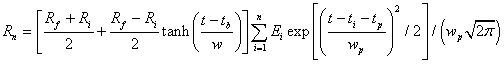,

where Ei is the amount extracted in year ti .

This spreads out the recycling of the extraction over a larger span of years than the extraction span. Thus, the mineral is spread out in time as well as in space as it is used to make items of use to humans.

The first recycling of a mineral will also be recycled a second time where Ei is the amount originally recycled in year ti , which further spreads it out over time according to the recycling function, as well as further spreading it out over space.

The second recycing will be recycled a third time. This repetition continues until the spread in time is so large that it contributes a negligible amount to the useable material. Thus, extraction and recycling of a mineral takes a space and time concentrated mineral and spreads it out over the entire space of the Earth and over time until it is impossible to further use that mineral to make items of use to humans. When that happens for all nonrenewable material, humans will only be able to use renewable materials, i.e., materials that grow because of solar energy.

To use this recycling theory for a given mineral, one has to make reasonable guesses about the values to use for the six parameters (Rf, Ri, tb, w, tp, wp) for that mineral. One way to confine some of the parameters is to fit the parameters to the known recycling rate for specific years, defined as the percent of consumption that is recyled material. This recycling rate is only known for the United States; so it is assumed to be representative of the world.

## Recycling of world minerals:

The following are recycling calculations for many minerals using reasonable guesses for the recycling parameters for each one:

 Iron-Ore Depletion & Recycling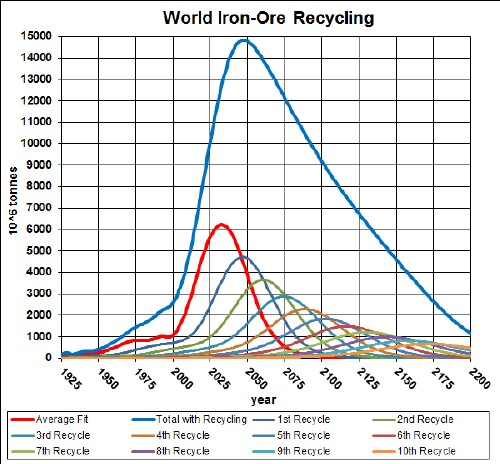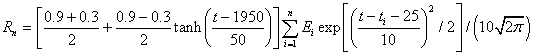The parameters in the recycling equation above were determined by fitting the iron/steel recycling data for the United States, thereby assuming that the world recycling rate was similar: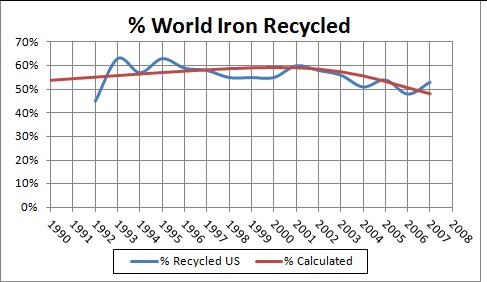The recycling data are the ratio of recycled material to consumed material, rather than recycled material to extracted material. So, they are only an approximation to the real yearly recycling rate, since it does not account for imported material into the US. Also, the ratio of recycled material to extracted material is not what is referred to as "recycling rate" in the equations; the equation rates are the ratio of recycled material to the extracted material in the same year in the past.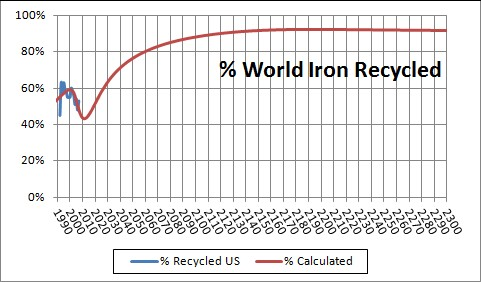Back to the top Bauxite Depletion & RecyclingThe parameters in the recycling equation above were determined by fitting the aluminum recycling data for the United States, thereby assuming that the world recycling rate was similar:The recycling data are the ratio of recycled material to consumed material, rather than recycled material to extracted material. So, they are only an approximation to the real yearly recycling rate, since it does not account for imported material into the US. Also, the ratio of recycled material to extracted material is not what is referred to as "recycling rate" in the equations; the equation rates are the ratio of recycled material to the extracted material in the same year in the past.Back to the top Copper Depletion & RecyclingThe parameters in the recycling equation above were determined by fitting the copper recycling data for the United States, thereby assuming that the world recycling rate was similar:The recycling data are the ratio of recycled material to consumed material, rather than recycled material to extracted material. So, they are only an approximation to the real yearly recycling rate, since it does not account for imported material into the US. Also, the ratio of recycled material to extracted material is not what is referred to as "recycling rate" in the equations; the equation rates are the ratio of recycled material to the extracted material in the same year in the past.Back to the top Lead Depletion & Recycling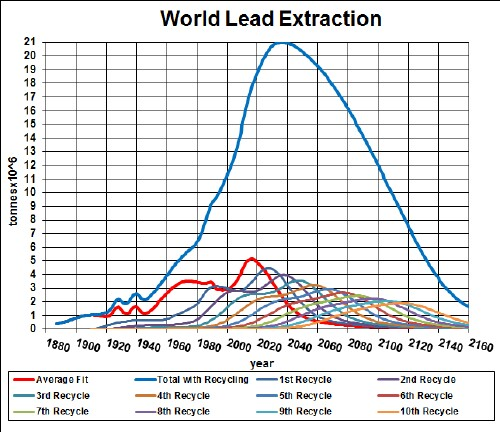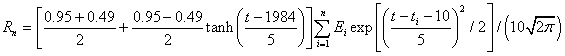The parameters in the recycling equation above were determined by fitting the lead recycling data for the United States, thereby assuming that the world recycling rate was similar: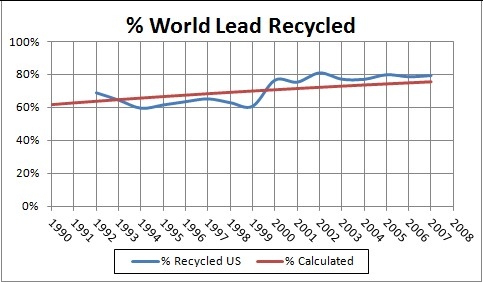The recycling data are the ratio of recycled material to consumed material, rather than recycled material to extracted material. So, they are only an approximation to the real yearly recycling rate, since it does not account for imported material into the US. Also, the ratio of recycled material to extracted material is not what is referred to as "recycling rate" in the equations; the equation rates are the ratio of recycled material to the extracted material in the same year in the past.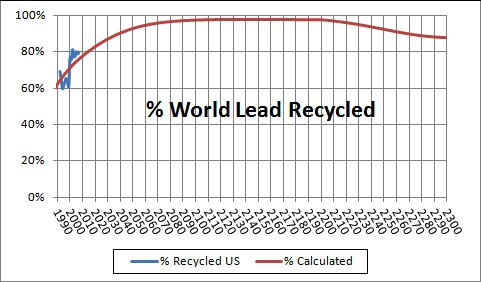Back to the top Nickel Depletion & Recycling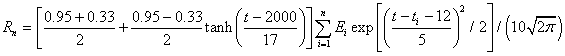The parameters in the recycling equation above were determined by fitting the nickel recycling data for the United States, thereby assuming that the world recycling rate was similar: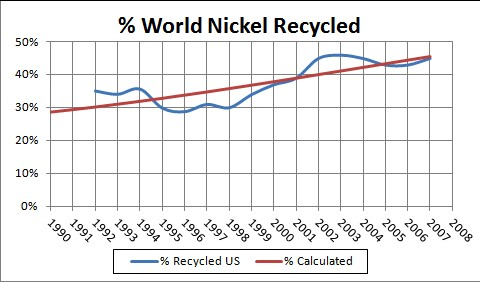The recycling data are the ratio of recycled material to consumed material, rather than recycled material to extracted material. So, they are only an approximation to the real yearly recycling rate, since it does not account for imported material into the US. Also, the ratio of recycled material to extracted material is not what is referred to as "recycling rate" in the equations; the equation rates are the ratio of recycled material to the extracted material in the same year in the past.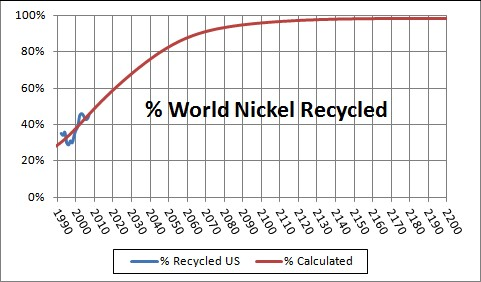Lithium Depletion & Recycling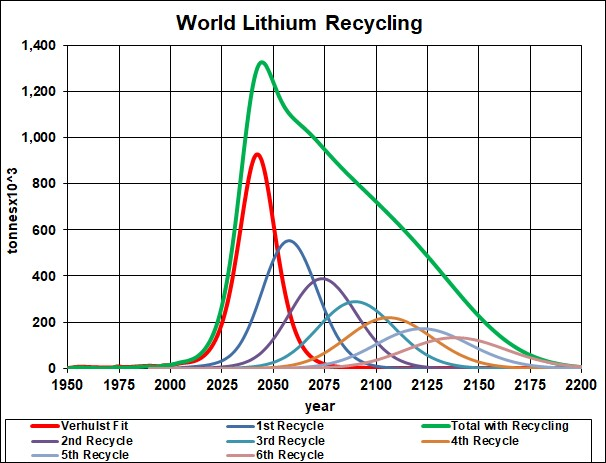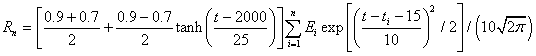Zinc Depletion & Recycling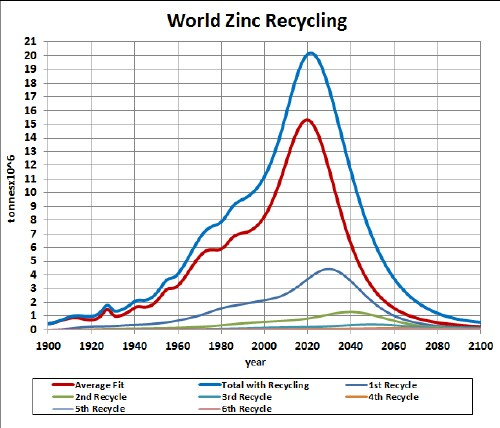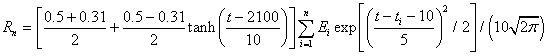The parameters in the recycling equation above were determined by fitting the zinc recycling data for the United States, thereby assuming that the world recycling rate was similar: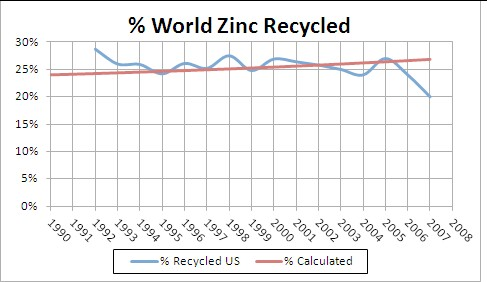The recycling data are the ratio of recycled material to consumed material, rather than recycled material to extracted material. So, they are only an approximation to the real yearly recycling rate, since it does not account for imported material into the US. Also, the ratio of recycled material to extracted material is not what is referred to as "recycling rate" in the equations; the equation rates are the ratio of recycled material to the extracted material in the same year in the past.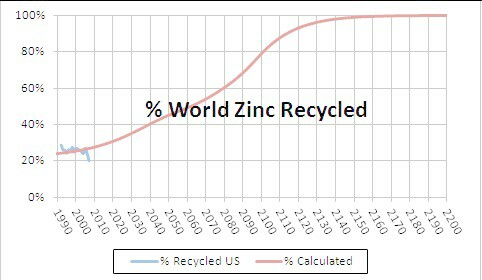Cadmium Depletion & RecyclingBack to the top Tin Depletion & Recycling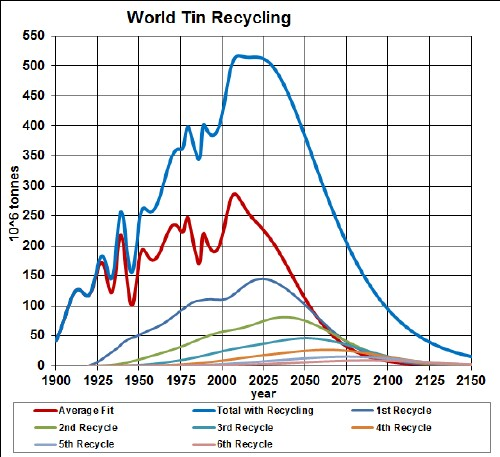The parameters in the recycling equation above were determined by fitting the tin recycling data for the United States, thereby assuming that the world recycling rate was similar: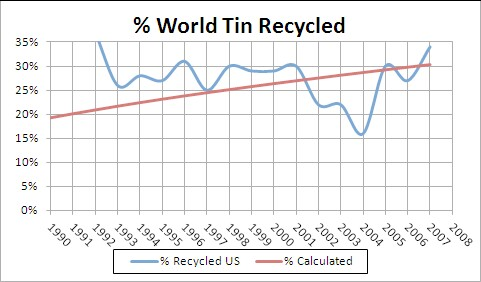The recycling data are the ratio of recycled material to consumed material, rather than recycled material to extracted material. So, they are only an approximation to the real yearly recycling rate, since it does not account for imported material into the US. Also, the ratio of recycled material to extracted material is not what is referred to as "recycling rate" in the equations; the equation rates are the ratio of recycled material to the extracted material in the same year in the past.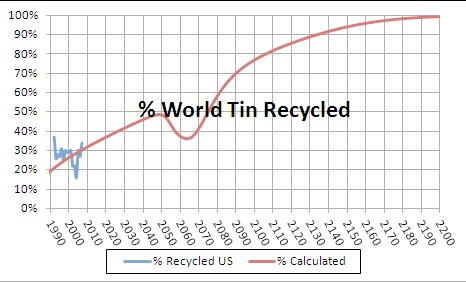Manganese Depletion & Recycling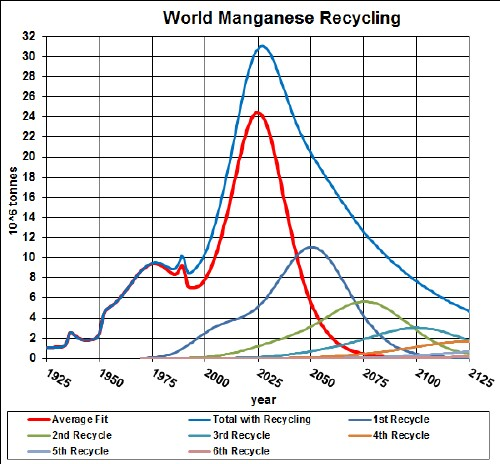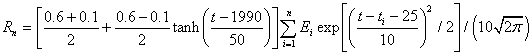Chromium Depletion & RecyclingThe parameters in the recycling equation above were determined by fitting the chromium recycling data for the United States, thereby assuming that the world recycling rate was similar:The recycling data are the ratio of recycled material to consumed material, rather than recycled material to extracted material. So, they are only an approximation to the real yearly recycling rate, since it does not account for imported material into the US. Also, the ratio of recycled material to extracted material is not what is referred to as "recycling rate" in the equations; the equation rates are the ratio of recycled material to the extracted material in the same year in the past.Back to the top Antimony Depletion & RecyclingVanadium Depletion & Recycling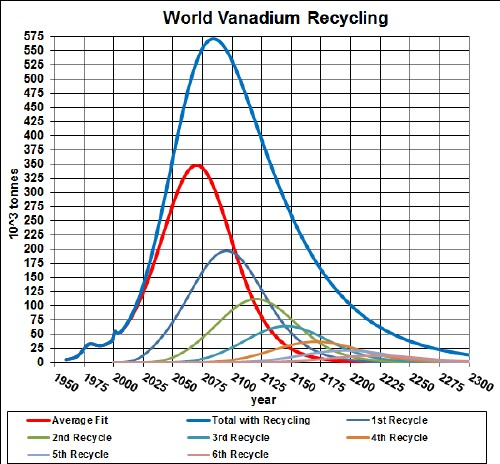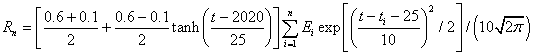Magnesium Depletion & Recycling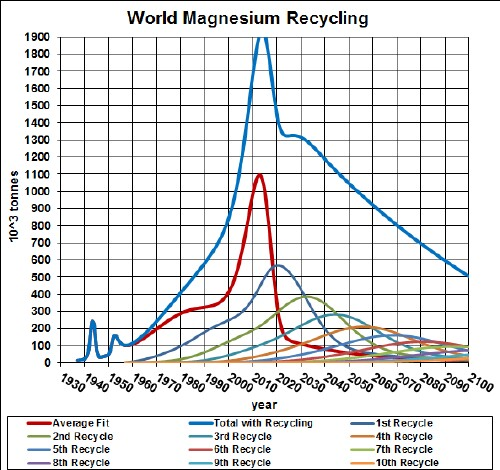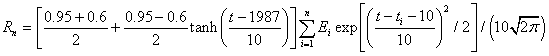The parameters in the recycling equation above were determined by fitting the magnesium recycling data for the United States, thereby assuming that the world recycling rate was similar: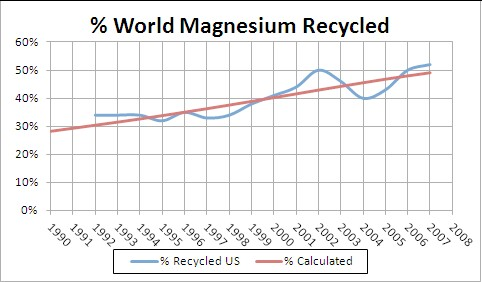The recycling data are the ratio of recycled material to consumed material, rather than recycled material to extracted material. So, they are only an approximation to the real yearly recycling rate, since it does not account for imported material into the US. Also, the ratio of recycled material to extracted material is not what is referred to as "recycling rate" in the equations; the equation rates are the ratio of recycled material to the extracted material in the same year in the past.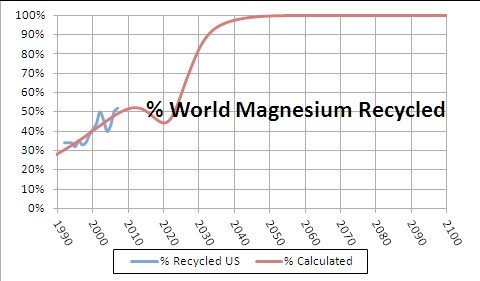Phosphate-Rock Depletion & Recycling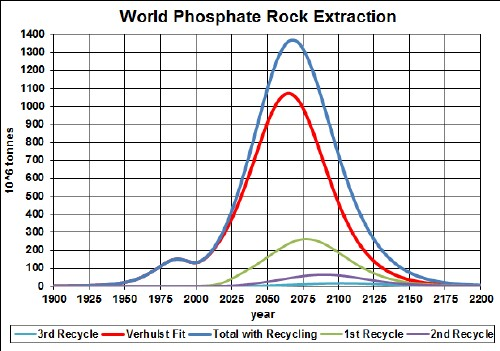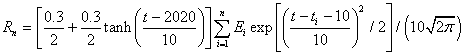Barite Depletion & RecyclingCobalt Depletion & RecyclingBack to the top Tungsten Depletion & Recycling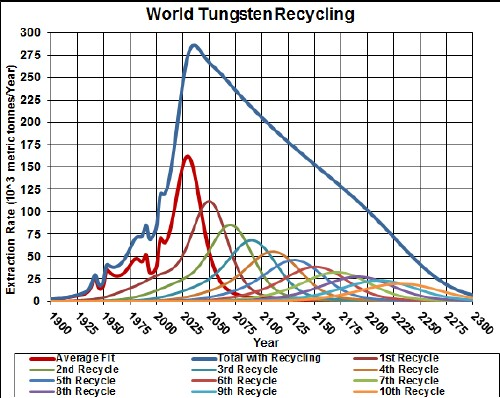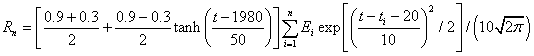Molybdenum Depletion & Recycling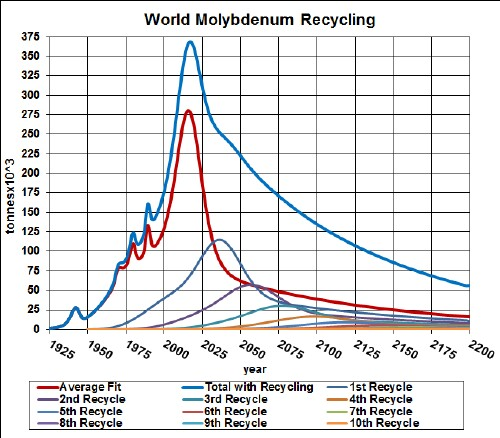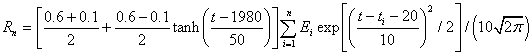Titanium Depletion & Recycling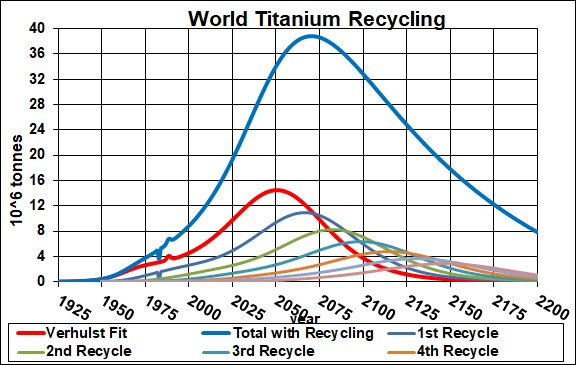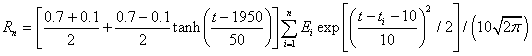The parameters in the recycling equation above were determined by fitting the titanium recycling data for the United States, thereby assuming that the world recycling rate was similar: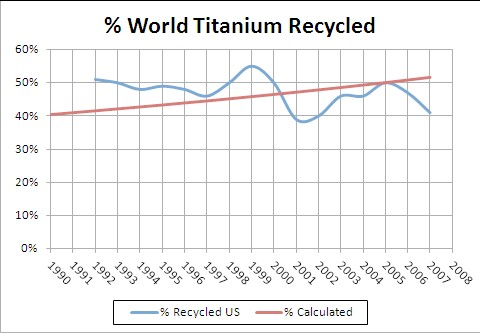The recycling data are the ratio of recycled material to consumed material, rather than recycled material to extracted material. So, they are only an approximation to the real yearly recycling rate, since it does not account for imported material into the US. Also, the ratio of recycled material to extracted material is not what is referred to as "recycling rate" in the equations; the equation rates are the ratio of recycled material to the extracted material in the same year in the past.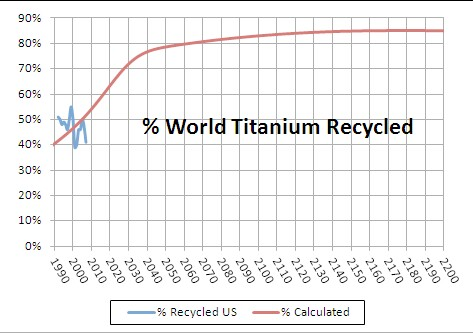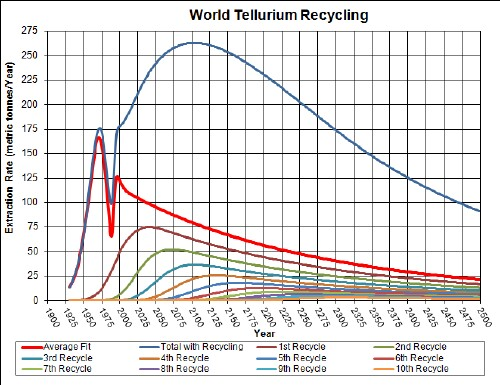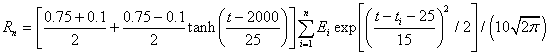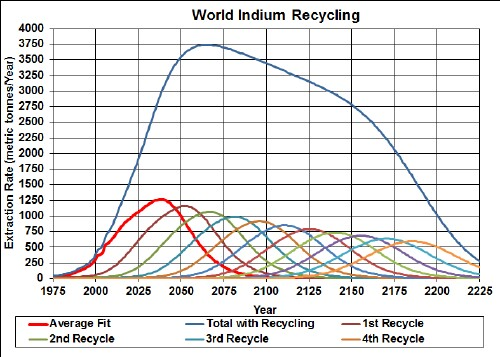Back to the top Gold Depletion & RecyclingSilver Depletion & RecyclingThese calculations can be easily redone in Excel by changing the fit parameters and the recycling parameters.